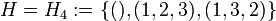# A3 in A4

This article is about a particular subgroup in a group, up to equivalence of subgroups (i.e., an isomorphism of groups that induces the corresponding isomorphism of subgroups). The subgroup is (up to isomorphism) alternating group:A3 and the group is (up to isomorphism) alternating group:A4 (see subgroup structure of alternating group:A4).
VIEW: Group-subgroup pairs with the same subgroup part | Group-subgroup pairs with the same group part | All pages on particular subgroups in groups

This article describes the subgroup$H$ in the group$G$. Here,$G$ is the alternating group:A4, acting on the set$\{ 1,2,3,4 \}$.$H$ is the subgroup:$\! H = H_4 := \{ (), (1,2,3), (1,3,2) \}$

It has three other conjugates:$\! H_1 := \{ (), (2,3,4), (2,4,3) \}, H_2 := \{ (), (1,3,4), (1,4,3) \}, H_3 := \{ (), (1,2,4), (1,4,2) \}$

With this notation, each$H_i$ is the stabilizer of$\{ i \}$ in$G$.

## Cosets

Each of the four subgroups has four left cosets and four right cosets. Further, for every pair of subgroups, there is exactly one coset that is a left coset for the first subgroup and a right coset for the second subgroup.

## Complements

All four subgroups have a unique common normal complement, which is the Klein four-subgroup of alternating group:A4:$\{ (), (1,2)(3,4), (1,3)(2,4), (1,4)(2,3) \}$

This is also the unique permutable complement to each of them.

Also, each of$H_1, H_2, H_3, H_4$ has each of the following three subgroups as a lattice complement that is not a permutable complement:$\{ (), (1,2)(3,4) \}, \qquad \{ (), (1,3)(2,4) \}, \qquad \{ (), (1,4)(2,3) \}$

### Properties related to complementation

Property Meaning Satisfied? Explanation Comment
permutably complemented subgroup has a permutable complement Yes See above
lattice-complemented subgroup has a lattice complement Yes See above
retract has a normal complement Yes See above
complemented normal subgroup normal subgroup with permutable complement No Not normal

## Arithmetic functions

Function Value Explanation
order of whole group 12
order of subgroup 3
index 4
size of conjugacy class 4
number of conjugacy classes in automorphism class 1

## Effect of subgroup operators

In the table below, we provide values specific to$H$.

Function Value as subgroup (descriptive) Value as subgroup (link) Value as group
normalizer the subgroup itself current page cyclic group:Z3
centralizer the subgroup itself current page cyclic group:Z3
normal core trivial subgroup -- trivial group
normal closure whole group -- alternating group:A4
characteristic core trivial subgroup -- trivial group
characteristic closure whole group -- alternating group:A4
commutator with whole group subgroup$\{ (), (1,2)(3,4), (1,3)(2,4), (1,4)(2,3) \}$ V4 in S4 Klein four-group

## Related subgroups

### Intermediate subgroups

The subgroup$H$ is a maximal subgroup of$G$, so there are no strictly intermediate subgroups between$H$ and$G$.

### Smaller subgroups

The subgroup$H$ is a group of prime order, so it has no proper nontrivial subgroup.

## Normality-related properties

For ease of reference, we take here the subgroup$H = \{ (), (1,2,3), (1,3,2) \}$, though the conclusions apply for the other three conjugates as well.

Some of the properties follow on account of being a Sylow subgroup.

Property Meaning Satisfied? Explanation Comment
normal subgroup equals its conjugate subgroups No
subnormal subgroup has a chain to whole group each normal in next No
pronormal subgroup Yes
automorph-conjugate subgroup all automorphic subgroups are conjugate subgroups Yes
isomorph-conjugate subgroup Yes
order-conjugate subgroup Yes
order-automorphic subgroup Yes
order-isomorphic subgroup Yes